# Graphing Trigonometric Functions The sine function Imagine a

• Slides: 12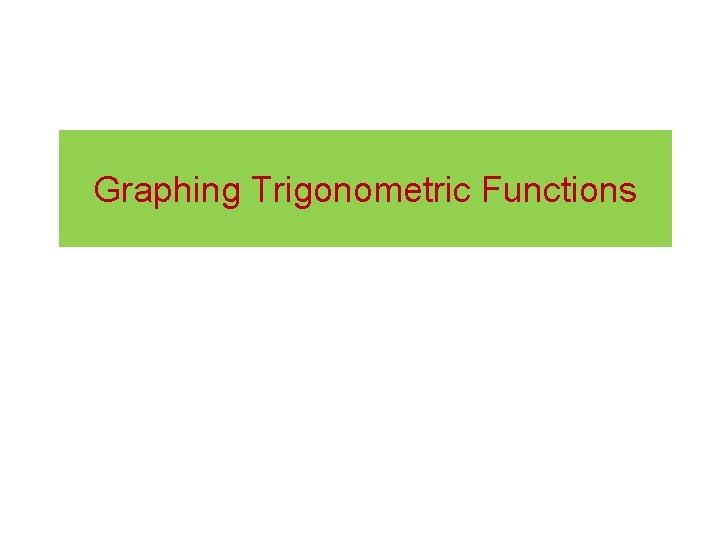Graphing Trigonometric Functions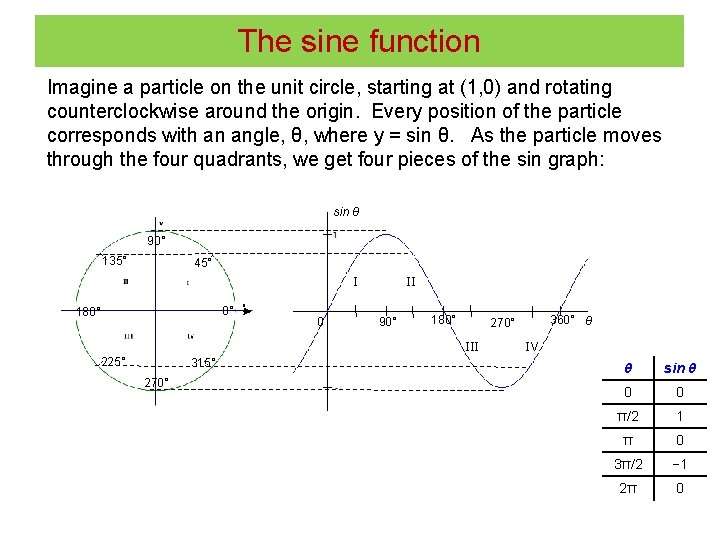The sine function Imagine a particle on the unit circle, starting at (1, 0) and rotating counterclockwise around the origin. Every position of the particle corresponds with an angle, θ, where y = sin θ. As the particle moves through the four quadrants, we get four pieces of the sin graph: sin θ 90° 135° 45° I 0° 180° 0 II 90° 180° III 225° 315° 270° 360° θ 270° IV θ sin θ 0 0 π/2 1 π 0 3π/2 − 1 2π 0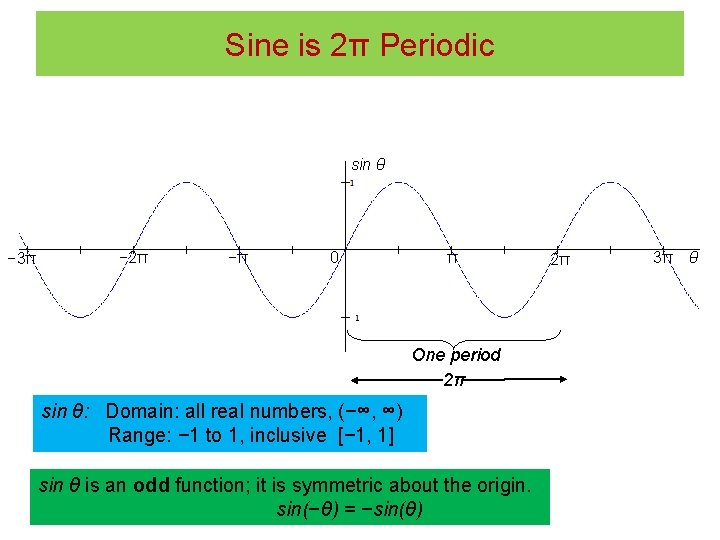Sine is 2π Periodic sin θ − 3π − 2π −π 0 π One period 2π sin θ: Domain: all real numbers, (−∞, ∞) Range: − 1 to 1, inclusive [− 1, 1] sin θ is an odd function; it is symmetric about the origin. sin(−θ) = −sin(θ) 2π 3π θThe cosine function Imagine a particle on the unit circle, starting at (1, 0) and rotating counterclockwise around the origin. Every position of the particle corresponds with an angle, θ, where x = cos θ. As the particle moves through the four quadrants, we get four pieces of the cos graph: cos θ 90° 135° 45° I 0° 180° 0 IV 90° II 225° 315° 270° 180° III θ 360° θ cos θ 0 1 π/2 0 π − 1 3π/2 0 2π 1Cosine is a 2π Periodic cos θ θ − 3π − 2π −π 0 π One period 2π cos θ: Domain: all real numbers, (−∞, ∞) Range: − 1 to 1, inclusive [− 1, 1] cos θ is an even function; it is symmetric about the y-axis. cos(−θ) = cos(θ) 2π 3πThe Tangent Function When cos θ = 0, tan θ is undefined. This occurs every odd multiple of π/2: { … −π/2, 3π/2, 5π/2, … } Table from θ = −π/2 to θ = π/2. Tanθ is π periodic. θ sin θ cos θ tan θ −π/2 − 1 0 −∞ −π/2 −∞ − 1 −π/4 − 1 0 0 0 1 π/4 1 ∞ π/2 ∞ −π/4 0 0 1 π/4 π/2 1 0Graph of Tangent Function: Periodic tan θ θ tan θ −π/2 −∞ −π/4 − 1 0 0 π/4 1 π/2 ∞ − 3π/2 −π/2 0 π/2 One period: π tan θ: Domain: θ ≠ π/2 + πn; i. e. , odd multiple of π/2. Range: all real numbers (−∞, ∞) tan θ is an odd function; it is symmetric about the origin. tan(−θ) = −tan(θ) Vertical asymptotes where cos θ = 0 3π/2 θ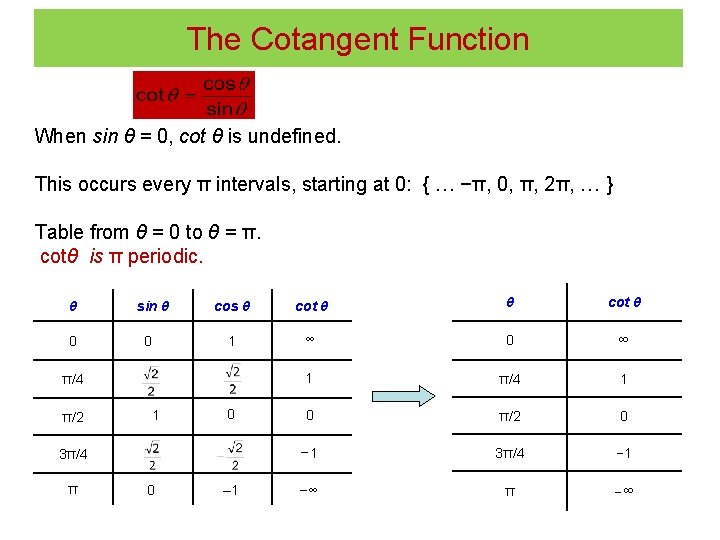The Cotangent Function When sin θ = 0, cot θ is undefined. This occurs every π intervals, starting at 0: { … −π, 0, π, 2π, … } Table from θ = 0 to θ = π. cotθ is π periodic. θ 0 sin θ 0 cos θ cot θ 1 ∞ 0 ∞ 1 π/4 1 0 π/2 0 − 1 3π/4 − 1 −∞ π/4 π/2 1 0 3π/4 π 0 – 1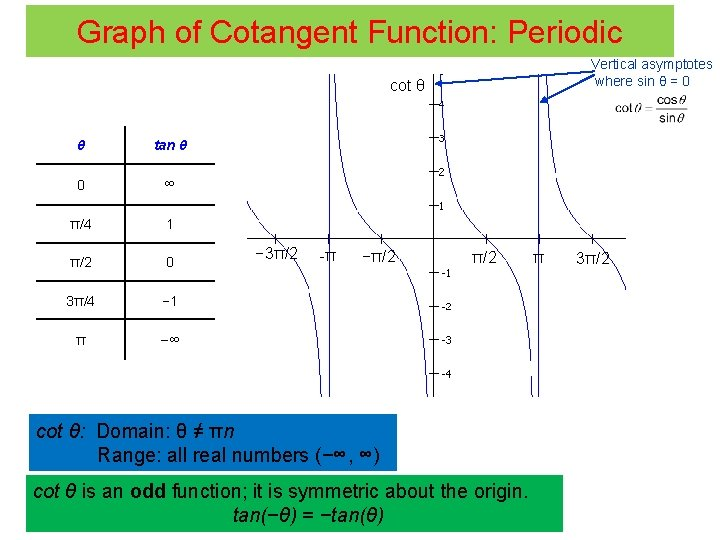Graph of Cotangent Function: Periodic Vertical asymptotes where sin θ = 0 cot θ θ tan θ 0 ∞ π/4 1 π/2 0 3π/4 − 1 π −∞ − 3π/2 -π −π/2 cot θ: Domain: θ ≠ πn Range: all real numbers (−∞, ∞) cot θ is an odd function; it is symmetric about the origin. tan(−θ) = −tan(θ) π 3π/2Cosecant is the reciprocal of sine csc θ − 3π Vertical asymptotes where sin θ = 0 θ 0 − 2π −π π 2π 3π sin θ One period: 2π sin θ: Domain: (−∞, ∞) Range: [− 1, 1] sin θ and csc θ: Domain: θ ≠ πn are odd (where sin θ = 0) (symmetric about the origin) Range: |csc θ| ≥ 1 or (−∞, − 1] U [1, ∞]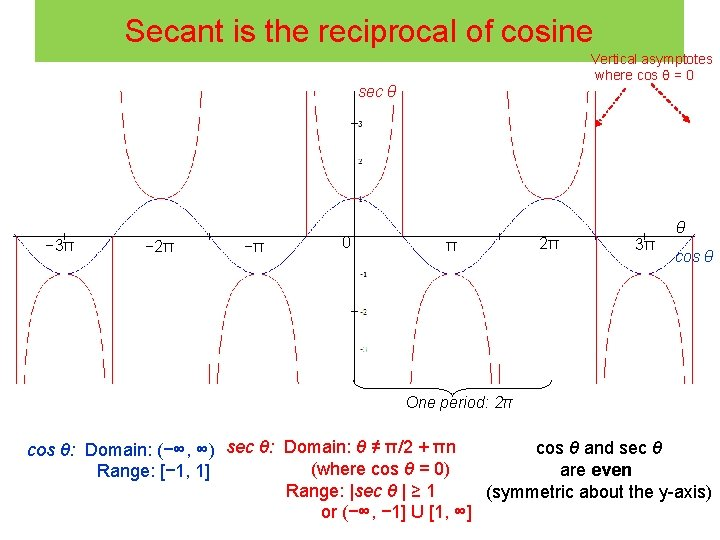Secant is the reciprocal of cosine Vertical asymptotes where cos θ = 0 sec θ − 3π − 2π −π 0 π 2π θ 3π cos θ One period: 2π cos θ and sec θ cos θ: Domain: (−∞, ∞) sec θ: Domain: θ ≠ π/2 + πn (where cos θ = 0) are even Range: [− 1, 1] Range: |sec θ | ≥ 1 (symmetric about the y-axis) or (−∞, − 1] U [1, ∞]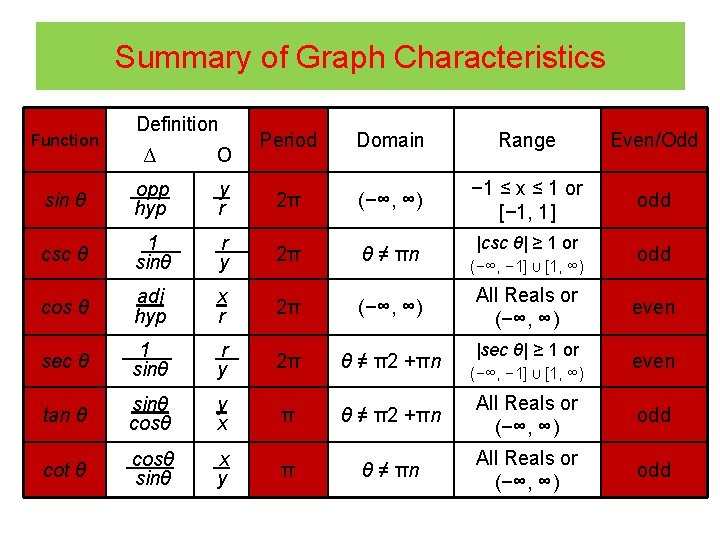Summary of Graph Characteristics Function Definition Period Domain Range Even/Odd − 1 ≤ x ≤ 1 or [− 1, 1] odd ∆ о sin θ opp hyp y r 2π (−∞, ∞) csc θ 1. sinθ r. y 2π θ ≠ πn cos θ adj hyp x r 2π (−∞, ∞) sec θ 1. sinθ r y 2π θ ≠ π2 +πn tan θ sinθ cosθ y x π θ ≠ π2 +πn All Reals or (−∞, ∞) odd cot θ cosθ. sinθ x y π θ ≠ πn All Reals or (−∞, ∞) odd |csc θ| ≥ 1 or (−∞, − 1] U [1, ∞) All Reals or (−∞, ∞) |sec θ| ≥ 1 or (−∞, − 1] U [1, ∞) odd even